# Review: Mathematical socks 2

More sartorial inquisition for your feetTwo years ago, I received some mathematical socks for Christmas. Following the unquestionable success of the mathematical review I conducted upon them, I have been sent more socks to review. How will these stack up mathematically? Did I get complements at work?

## UnboxingMathematical socks as you might expect to find them on a Christmas tree

The socks come neatly folded in half, with a black plastic tag connecting the top and toe to a card label. The label describes them as ‘rather clever socks’, and backs this up by noting that they are made of 75% cotton, 23% nylon and 2% elastane. This does indeed add up to 100%: clever socks.

The presentation shows off a bold white-on-black design, reminiscent of mathematics written on a blackboard. We do like chalk here at Chalkdust, and the equations placed front and centre certainly look suitably mathematical. More on that later.

Pulling on the label neatly snaps the plastic tag, and I found that I was able to go from packaged to foot in under 12 seconds.

A note about folding in half: Marie Kondo has explicitly taught us the importance of folding in thirds, so the packaging loses a sixth of a point for this error.

## Mathematical content

In contrast to mathematical socks 1, these display different formulae on each sock. For clarity, I have photoshopped together the individual sides of the socks. Together, there are an amazing sixteen equations! Imagine you had to pick the sixteen most important expressions in mathematics to place on a sock. Would you have picked these?#### 1. Pythagoras returns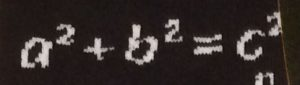The undisputed king of mathematical equations. This rightly takes it place at the top of the sock.

#### 2. Binomial expansion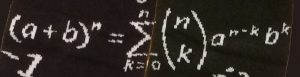Who remembers writing these out in full in A-level? Now you get to relive the experience on your feet!

#### 3. Imaginary number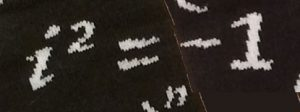Three equations in, and we’ve already hit complex numbers. A bit of a learning curve, but necessary for some of the forthcoming expressions.

#### 4 & 5. Battle of: Definition of e

One for each sock! Do you put your limits on your left?And your sums on your right?Or the other way round? Let us know in the comments.

#### 6. Fourier transform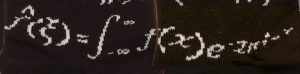Now we head into the realm of formulae cut off by the heel of the sock. Good to see the definition of the Fourier transform here,$$\hat{f}(\xi) = \int_{-\infty}^\infty f(x) \mathrm{e}^{-2\mathrm{\pi i}x\xi} \, \mathrm{d}x,$$and we should appreciate the embroidery of everyone’s favourite Greek letter, $\xi$, but how do we feel about the choice to leave out the $1/\sqrt{2\pi}$?

#### 7. Error function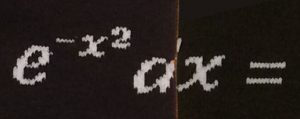Quite a lot missing from this one, I think, but what we’re seeing is the heart of the error function,$$\frac{2}{\sqrt{\pi}}\int_0^z \mathrm{e}^{-x^2}\,\mathrm{d}x = \mathrm{erf}(z).$$Admittedly the choice of $x$ as dummy variable is a little unusual.

#### 8. Definition of differentiationPretty usual to see this with $t$ instead of $x$ but we’ll accept,$$f'(t) = \lim_{h\to0}\frac{f(t+h)-f(t)}{h}.$$

#### 9. Riemann zeta functionA lot has been cut off here but I think it’s safe to say we’re seeing the definition of the function we most like to make hypotheses about,$$\zeta(s) = \sum_{n=1}^\infty \frac{1}{n^s}.$$

#### 10 & 11. Battle of: Euler’s formula

Vote now: the most boring equation in all of mathematics,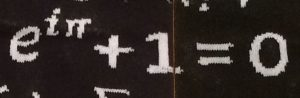or an interesting relation between the number of faces, vertices, edges of convex polyhedra,But are socks really convex polyhedra? What is the Euler characteristic of a sock?

#### 12. Second Chebyshev function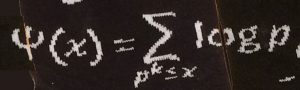What a find! I can’t believe this found its way onto a sock by accident. You might know Chebyshev from the famous Matlab package, Chebfun. But here it’s not Chebyshev polynomials we’re talking about, but instead one of two related formulae named after him: this one gives the sum extending over all prime powers less than or equal to $x$:$$\psi(x) = \sum_{p^k \leq x}\log p.$$But even more exciting is the version where $k=1$, since it’s normally named $\vartheta(x)$: yes, vartheta!

#### 13. Normal distribution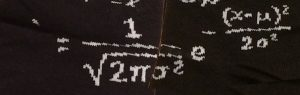You have to admire the typesetting required to get all this onto a sock, and indeed they’ve only managed to put on the right-hand side. But still! It’s the probability density of the normal distribution,$$f(x \mid \mu, \sigma^2) = \frac{1}{\sqrt{2\pi\sigma^2} } \mathrm{e}^{ -\frac{(x-\mu)^2}{2\sigma^2} }.$$

#### 14. Asymptotic law of distribution of prime numbers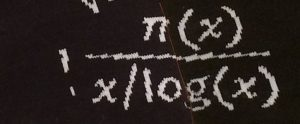Another dip into the primes, as we find the limiting behaviour for the distribution of primes. The full story was in Chalkdust Issue 03, but here we see that the number of prime numbers less than or equal to $x$, given by $\pi(x)$, has the behaviour$$\lim_{x\to\infty}\frac{\pi(x)}{x/\log(x)} = 1.$$Very cool.

#### 15. Log rules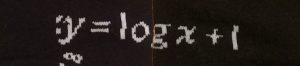A little trivial, this one, but important nonetheless.$$\log xy = \log x + \log y$$

#### 16. Basel problemAnother expression proved by Euler (whose hometown was Basel), and now featuring in all part (d)s of questions about Fourier series.$$\sum_{n=1}^\infty\frac{1}{n^2} = \frac\pi6$$

Sixteen excellent equations, featuring five sums, three limits and two integrals. Some essential results from the field of primes, trigonometry, statistics and analysis. There’s some overlap with Ian Stewart‘s seventeen equations that changed the world, but I’m pleased that there is an intentional move away from physics: how easy would it have been to throw in $E=mc^2$? Full marks for this selection.

## Typesetting

Typesetting on a sock is difficult, and mathematical socks 1 clearly struggled. But the text is larger here, allowing for more detail.

What I think we’re seeing is Microsoft Office typesetting combined with hand-drawn symbols (like $\sum$), with custom spacing so it fits better on the sock.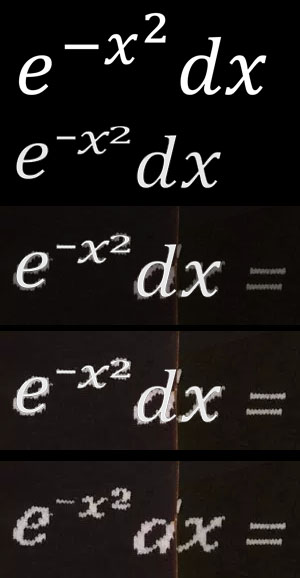Custom-spaced Cambria Math… what do you think?

What do you reckon? Take a look at the $\xi$ in particular in the Fourier transform.
Typesetting grade: C. Good effort but too much cut off.

## Fashion

Does black and white ever go out of style? Writing that goes all the way up the leg. Pair with sandals to really show off the lettering.

## Fit

The socks come in two sizes, and I found the larger size perfectly fine on size 9 feet. The large amount of internal stitching provides a slightly tight fit.

Unfortunately, the website that sells these describes the sizes as “4–7 UK (8–11 US) and 7–11 UK (10–12 US)”, demonstrating an unforgivably poor mathematical understanding of how to add 1…

## UtilityKyoto Garden in Holland Park

I took these socks for a trip round Holland Park, visiting the Kyoto Garden. The garden was lovely but I worry that the socks scared off the koi, as they were missing in action.

## Overall review

Avoid awkward first date moments by whipping off your shoes and debating which of the sixteen equations is your favourite.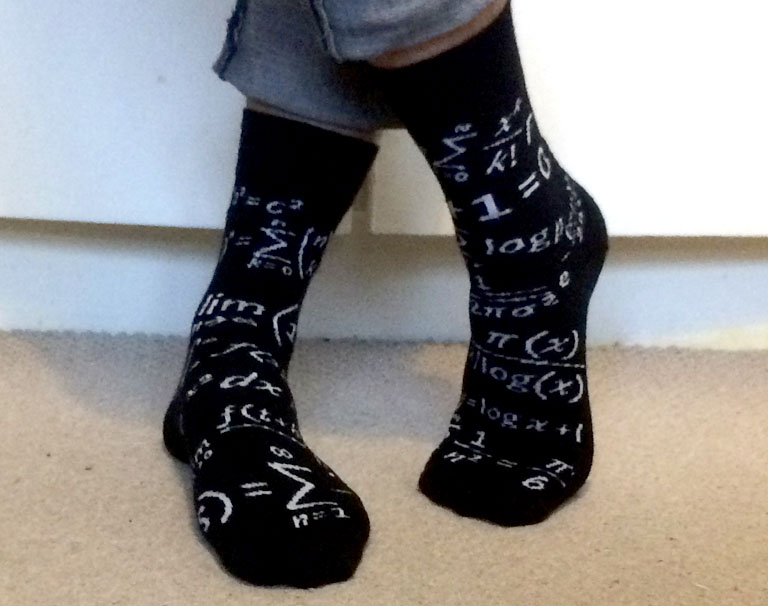Also niftyAdam is an assistant professor at Durham University, where he investigates weird, non-Newtonian fluids. If he’s not talking about the maths of chocolate fountains he is probably thinking about fonts, helping Professor Dirichlet answer your personal problems, and/or listening to BBC Radio 2.